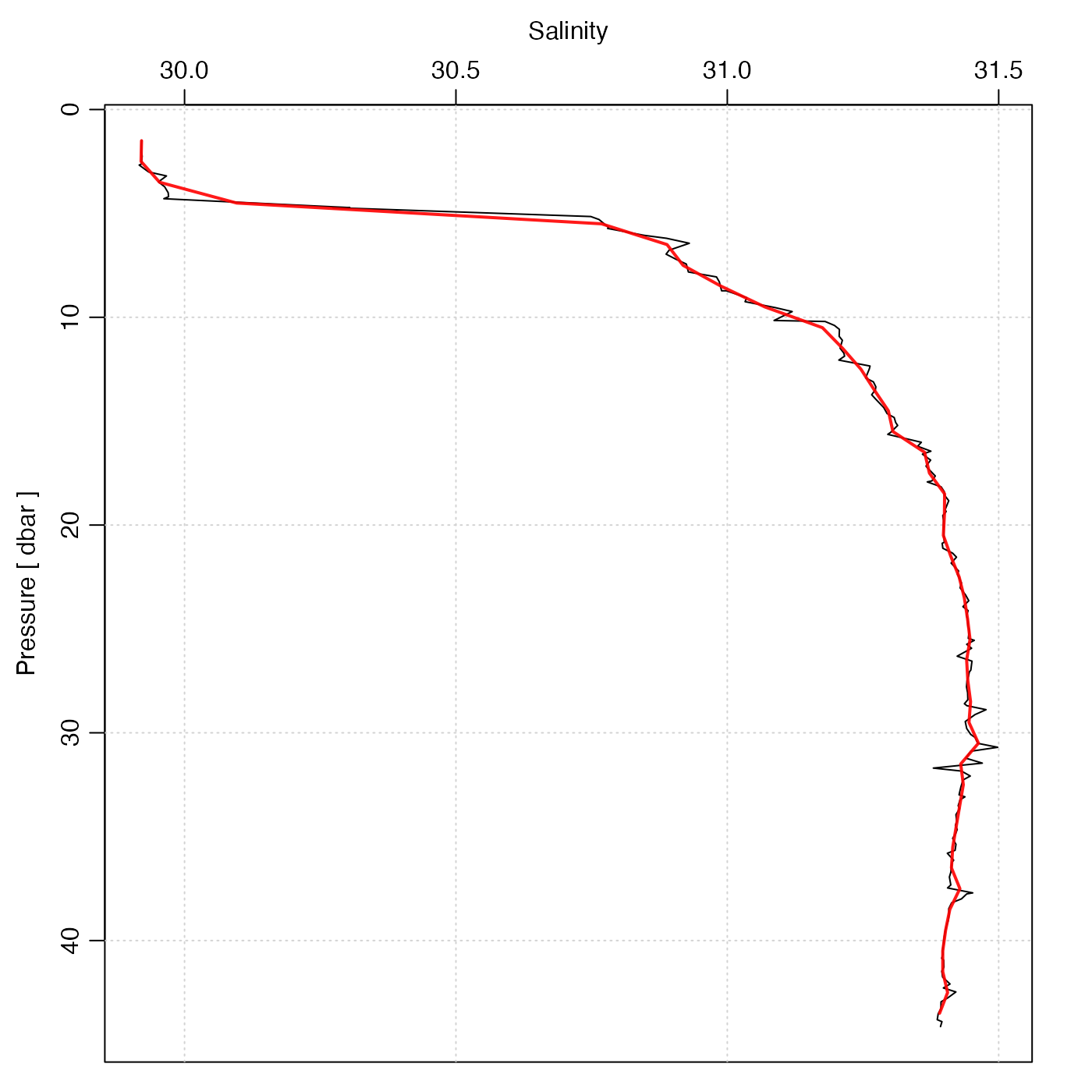The function FUN is applied to f in bins specified by xbreaks. (If FUN is mean(), consider using binMean2D() instead, since it should be faster.)

binApply1D(x, f, xbreaks, FUN, ...)

## Arguments

x a vector of numerical values. a vector of data to which the elements of FUN may be supplied values of x at the boundaries between bins; calculated using pretty() if not supplied. function to apply to the data arguments to pass to the function FUN

## Value

A list with the following elements: the breaks in x and y (xbreaks and ybreaks), the break mid-points (xmids and ymids), and a matrix containing the result of applying function FUN to f subsetted by these breaks.

Other bin-related functions: binApply2D(), binAverage(), binCount1D(), binCount2D(), binMean1D(), binMean2D()

## Examples

library(oce)
## salinity profile with median and quartile 1 and 3
data(ctd)
p <- ctd[["pressure"]]
S <- ctd[["salinity"]]
q1 <- binApply1D(p, S, pretty(p, 30), function(x) quantile(x, 1/4))
q3 <- binApply1D(p, S, pretty(p, 30), function(x) quantile(x, 3/4))
plotProfile(ctd, "salinity", col='gray', type='n')polygon(c(q1$result, rev(q3$result)),
c(q1$xmids, rev(q1$xmids)), col='gray')points(S, p, pch=20)